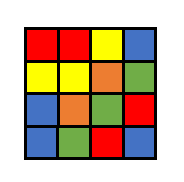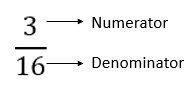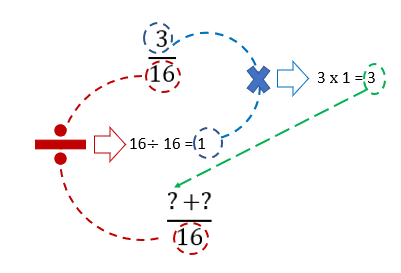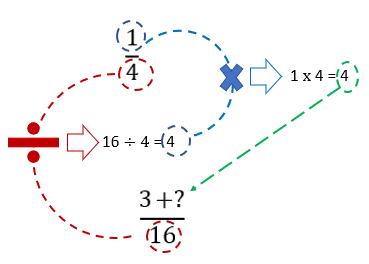# Adding Fractions

This guide shows you how to add fractions and includes how to add fractions with different denominators.

Fractions represent a part of a whole mathematically. They are a mathematical entity and mathematical operations like addition, subtraction, multiplication and division can be performed on them. In this article we will learn how to add fractions. Lets quickly revise the concept of fractions first.

Imagine one side of a magic cube as shown below. It is divided into small squares of different colours. What is the fraction of yellow squares?To determine the fraction, we need to count the yellow squares and the total number of squares. Yellow squares are 3 and total number of squares are 16 so the fraction of yellow squares is:We have labelled the numerator and denominator as well so that you may recall, incase you have forgotten, that the upper number of the fraction is called the numerator and the lower number of the fraction is called the denominator! Now let’s try writing another fraction: what is the fraction of red squares?

Yes, that’s right! It is . This fraction can be simplified and written as:

If you are not sure how to simplify fraction, see our detailed article on how to simplify fractions. Now, if you are asked what is the fraction of red and yellow squares combined, how would you answer? One way to do it is to count the total number of red and yellow squares and express them as a fraction.

Total red and yellow squares: 3 + 4 = 7

Fraction of combined red and yellow squares:

This means that if fraction of yellow square is added to the fraction of red squares the answer must be

We added those fractions by counting the squares and it helps in visualizing the concept of fraction addition but remember that most of the times when you are solving fractions, visual figures are not available and you have to work with just fractions. Let’s see how to add the fractions by following simple mathematical steps.

Suppose you don’t have the square available to consult and you are just asked to add and .

Step 1:

Find LCM (Least Common multiple) of denominators of both fractions. If you are not familiar with the concept of LCM please see our article on GCF and LCM. We will briefly outline here how to find LCM as well in mini steps:

Mini-Step 1.1: List prime factors of both numbers

Mini-Step 1.2: Write the factors in index form i.e. when a factor occurs more than once, write it raised to some power. For example, when 2 is multiplied four times it can be written as 2 raised to power 4: . When a number has no power, it means its power is 1.

Mini-Step 1.3: Choose maximum power of each factor of both numbers and multiply them to get LCM. If a factor occurs in case of only one number it is always chosen, if a factor occurs in both numbers, its maximum power is chosen.

Step 2:

Once LCM is calculated, write it in the denominator.

Step 3:

Here comes the tricky part, pay attention!

For numerator, you have to write sum of two numbers.

For first number, divide the denominator of first fraction by the LCM and multiply the answer by the numerator of the first fraction. Confusing? See the figure below and re-read the statement to get better understanding.For second number of numerator sum, repeat the same procedure considering the second fraction. Divide LCM (16) by denominator of second fraction (4) and multiply the answer (164 = 4) by numerator of second fraction (14 = 4).So we have:

That is the same fraction that we got by visually counting the boxes!

Let’s do another example where one fraction is a proper fraction (numerator smaller than denominator) and the second one is an improper fraction (numerator is greater than denominator). The method of addition is the same and it does not matter whether the fraction is proper or improper.

Add:

Try to do it yourself before going through the solution.

Step 1:

Find LCM

Mini-Step 1.1:

Mini-Step 1.2:

Mini-Step 1.3: Factors 1 and 3 occur for both numbers so their maximum power is chosen. Factor 5 occurs only for 15 and not for 9 but it also chosen, because as we mentioned earlier: if a factor occurs for only one number it is always chosen.

Step 2:

Once LCM is calculated, write it in the denominator.

Step 3:

Since two fractions are being summed, numerator will be a sum of two numbers. First number is obtained by the following procedure:

• Divide LCM by denominator of first fraction:
• Multiply the answer by numerator of first fraction:

So,

Repeat same procedure by considering second fraction.

Finally, we have:

The final answer can be simplified further to . If you are not sure how to simplify fractions, see our article on simplifying fractions.

Adding more than two fractions

At this point in the article, hopefully you have mastered the art of adding two fractions. If you have to add more than two fractions, the procedure is the same. Let’s do an example to illustrate it.

Add:

Step 1:

Find LCM

Mini-Step 1.1:

Mini-Step 1.2:

Mini-Step 1.3: Choose highest powers of factors for LCM.

Step 2:

Once LCM is calculated, write it in the denominator.

Step 3:

Since three fractions are being summed, numerator will be a sum of three numbers. First number is obtained by the following steps:

• Divide LCM by denominator of first fraction:
• Multiply the answer by numerator of first fraction:

So,

Repeat same procedure by considering second fraction.

This gives us,

Again, repeat the same procedure by considering the third fraction.

Finally, we have:

The final answer can be further simplified:

So, the final answer of addition of the three fractions is

Adding fraction and integers

An important concept while learning the addition of fractions is the addition of fractions and integers. Integers are simple numbers. In a lot of situations, you might need to add fractions to integers and it is pretty easy and simple. Let us show you how.

The single most important thing that you need to learn in order to perform this addition is: integers are also fractions with denominator equal to 1! Here are some examples to help you understand what we are talking about:

Using this information, now you can treat integers as fractions and add them to fractions using the same procedure as outlined above.

Add:

This question can be rewritten as:

Step 1:

Find LCM

Mini-Step 1.1:

Mini-Step 1.2: There are no factors with powers. 1 always remains 1 no matter its power.

Mini-Step 1.3:

Step 2:

Once LCM is calculated, write it in the denominator.

Step 3:

Since two fractions are being summed, numerator will be a sum of two numbers. First number is obtained by doing the following steps

• Divide LCM by denominator of first fraction:
• Multiply the answer by numerator of first fraction:

So,

Repeat same procedure by considering second fraction.

Finally, we have:

Final Thoughts!

In this article we learned how to add fractions and how to add integers to fractions. This is a pretty useful skill to master and lays down foundation of some advanced mathematical work. Next, we will talk about subtracting fractions. Check out our website for other interesting and useful mathematical concepts explained in an easy way! Want to have a shortcut? Try our fraction calculator.